Do you like the article?
Share it with others -

Use new possibilities of MetaTrader 5

# Practical Implementation of Digital Filters in MQL5 for Beginners

25 March 2010, 08:33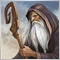0
13 155

### Introduction

So, in my previous article I've made a code analysis of simple indicator and slightly covered the interaction of this indicator with MetaTrader 5 Client Terminal. Now, before we go any further, we should take a closer look at the results of expert compilation in "Errors" tab of "Toolbox" window in MetaEditor. From here you can begin further study of SMA indicator's code, that I had proposed earlier.

### Indicator compilation errors

In our situation, when compiling any of two versions of the code, in case of no changes the compilation process is quite smooth with the expected result: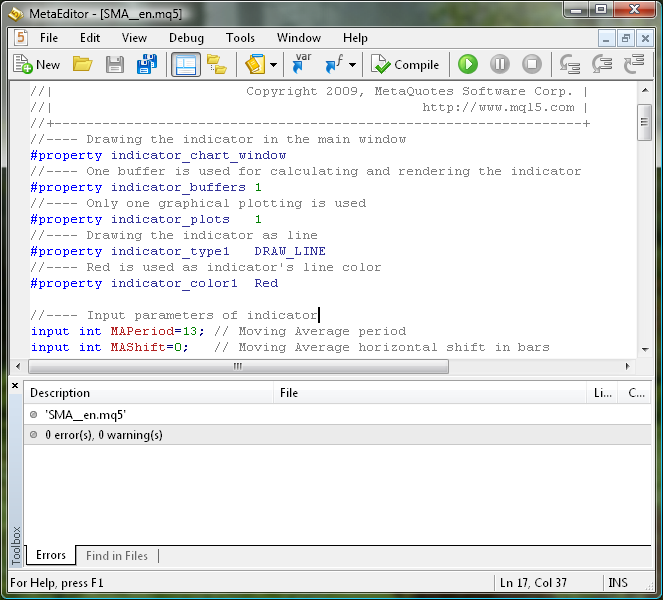There are no errors, and along with indicator file with .mq5 extension appeared the similar file with .ex5 extension.

Typically, when you work with the code you can't evade errors. They are regularly made by programmers. For this purpose MetaEditor has a built-in mechanism for checking the compiled code for all sorts of errors, and when it finds them it will give a full list of generated errors.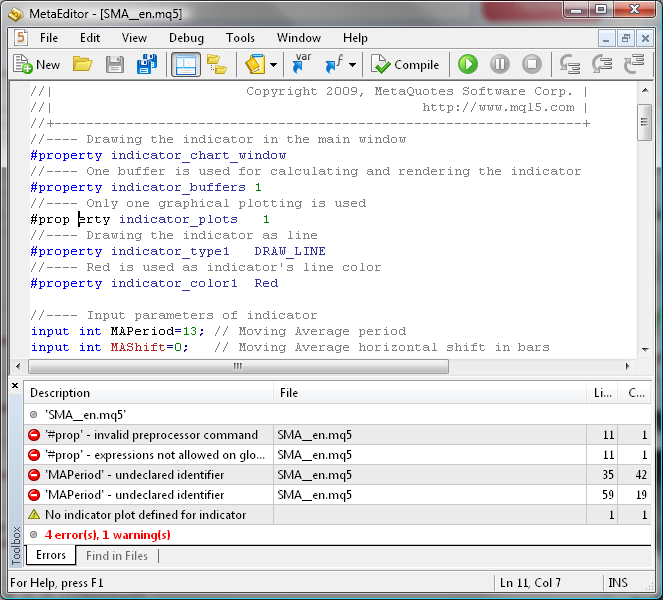To detect the location of an error you can just double-click on the appropriate line with the contents of the error in the "Toolbox" window. The compiler in most cases will accurately indicate the line of code where the error was found, using appropriate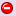icon.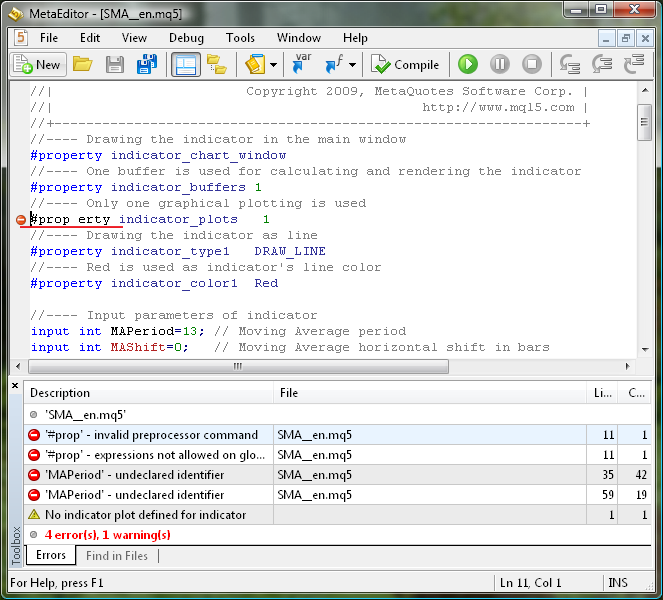You should consider one thing. One error in the code can generate a whole sequence of compilation errors. So, in order to remove the sequence of errors, it's sufficient to go to first line where compiler has found an error, and to correct the code. Quite naturally, there can be a lot of such compilation error sequences. So, after fixing one error in the code we must recompile it again, and if the compiler finds errors, then we must look for the first line in "Errors" tab of "Toolbox" window: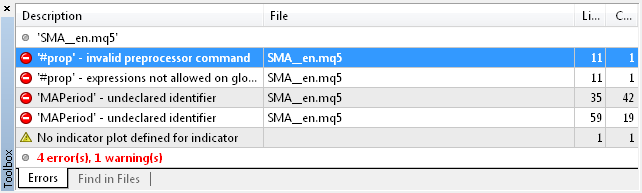Perhaps the most effective method of comprehending this will be meaningful, destructive impact on our code in order to study how compiler will react on consciously made errors. The technique is quite simple - do the error in particular part of code, press "Compile" button in MetaEditor and watch the result of compilation. It will be even better if you intuitively remember such result of destructive impact on the code. In any case this can be useful in further practice, when working with MQL5 code.

Here is the list of possible destructive changes in indicator's source code:

1. Making a space in any operator or variable.
2. Clearing a semicolon ";" mark.
3. Adding a ";" mark in different parts of code.
4. Deleting an operator.
5. Removing or adding a brace or a parenthesis.
6. Removing a comma "," mark.
7. Adding an extra input parameter in OnCalculate() function.
8. Dividing a variable by zero.
9. Replacing a "==" mark to "=" in "if" operator line.
10. Changing direction of increment in a variable from bar++ to bar--.

Naturally, the compiler doesn't always find the place with an error right where it is made. That's why this preliminary work is worth doing in order to understand how to deal with such situations. Well, one more explanation regarding errors - MetaEditor compiler only determines the errors of the MQL5 language itself, and in most cases it doesn't find logical errors of programming!

If you listen to any particular individual, then, with all the richness of any human language, it turns out, that he uses only a small portion of tools, that express his thoughts and needs. In most situations, it turns out that actual used vocabulary is significantly smaller than available. The same principle can be applied to MQL5. At first, while mastering the MQL5 language, you should get accustomed to the most commonly used operators and expressions of this programming language. And as you learn this language, you can gradually expand the boundaries of your actual vocabulary.

For example, you can use four types of variables (int, double, bool, string), if-else conditional operator, for loop operator, {} compound operator and return operator. You should also thoroughly learn how to use a semicolon ";" and a comma ",". Perhaps it would be wise to learn mathematical and trigonometric functions. These tools are more than enough for training and practicing your initial programming skills!

### Further indicator refinement

MQL5 capabilities of refining indicator, displayed in MetaTrader Client Terminal, are fairly simple and standard. They consist of global level operators:

```//---- Indicator's author
#property copyright "2010, MetaQuotes Software Corp."
//---- Indicator version number
#property version   "1.00"
//---- Drawing the indicator in the main window
#property indicator_chart_window
//---- One buffer is used for calculating and drawing the indicator
#property indicator_buffers 1
//---- Only one graphical plotting is used
#property indicator_plots   1
//---- Drawing the indicator as line
#property indicator_type1   DRAW_LINE
//---- Red is used as indicator's line color
#property indicator_color1  Red
//---- Indicator line is continuous curve
#property indicator_style1  STYLE_SOLID
//---- Indicator line thickness is equal to 1
#property indicator_width1  1
//---- Displaying indicator's label
#property indicator_label1  "SMA"```

And of function calls of OnInit():

```//---- Variable initialization for indicator's short name
string shortname;
StringConcatenate(shortname,"FATL(",FATLShift,")");
//--- Creating labels to display in Data Window
PlotIndexSetString(0,PLOT_LABEL,shortname);
//--- Creating name to display in a separate window and in tool-tip
IndicatorSetString(INDICATOR_SHORTNAME,shortname);
//--- Defining accuracy of displaying indicator's values
IndicatorSetInteger(INDICATOR_DIGITS,_Digits+1);
//--- Prohibition of displaying blank values
PlotIndexSetDouble(0,PLOT_EMPTY_VALUE,0.0);```
The StringConcatenate() function assembles the indicator name's string using this formula:
`   shortname = shortname + "SMA(" + MAPeriod + "," + MAShift + ")";`

According to recommendations in Applying One Indicator to Another article, it wouldn't hurt to add PlotIndexSetInteger() function call into OnCalculate():

```//---- Calculating the 'first' starting number for the bars recalculation loop
if(prev_calculated==0)        // Checking the first start of the indicator calculation
{
first=FATLPeriod-1+begin;  // Starting number for calculation of all bars
//--- Increasing the start of data position by 'begin' bars, because the
//    calculations are based on data of another indicator
if(begin>0)
PlotIndexSetInteger(0,PLOT_DRAW_BEGIN,begin+FATLPeriod);
}
else first=prev_calculated-1; // Starting number for calculation of new bars```
It is natural, that after inclusion of these additional lines of code, our indicator slightly increased in size and became a little bit more complicated, but now it has more user-friendly interface.

### The result of the previous work as a template for creating new indicators

All this is certainly interesting, but there is one quite natural question - why to invent the wheel and to repeat the code of indicator, which is already available in Client Terminal in two versions? In the form of Moving Average.mq5  technical indicator and Custom Moving Average.mq5  custom indicator. The answer is simple. To learn how to quickly write code of similar indicators, just by using my previously proposed SMA indicator code as a template, thus saving your intellectual resources as much as possible! For example, you can try to write code in MQL5 for a digital filter, such as FATL from Finware.

In general, the formula for calculating the digital filter is:

FILTER = SUM (K(i) * CLOSE (i), FilterPeriod)

where:

• SUM — the sum.
• K(i) — the weighting coefficient.
• CLOSE (i) — the Close price of current bar.
• FilterPeriod — the number of bars for averaging.

This formula doesn't differ much from SMA indicator formula:

SMA = SUM ((1 / MAPeriod ) * CLOSE (i), MAPeriod)

The difference is that the period, on which calculations with a digital filter are made, is strictly fixed and is individual for specific digital filter, as well as K(i) weighting coefficients. The weighting coefficients themselves and the digital filter period are calculated using specialized algorithms. Analyzing these algorithms is beyond the scope of this article, so we'll confine ourselves to use ready values for the FATL digital filter. Those, who are interested in idea of digital signal filtering, can visit Digital Methods Generator web-site (in Russian). The formula of a variant of FATL indicator in MQL4 is not a secret:

```     FATL =  0.4360409450 * Close[bar + 0]
+ 0.3658689069 * Close[bar + 1]
+ 0.2460452079 * Close[bar + 2]
+ 0.1104506886 * Close[bar + 3]
- 0.0054034585 * Close[bar + 4]
- 0.0760367731 * Close[bar + 5]
- 0.0933058722 * Close[bar + 6]
- 0.0670110374 * Close[bar + 7]
- 0.0190795053 * Close[bar + 8]
+ 0.0259609206 * Close[bar + 9]
+ 0.0502044896 * Close[bar + 10]
+ 0.0477818607 * Close[bar + 11]
+ 0.0249252327 * Close[bar + 12]
- 0.0047706151 * Close[bar + 13]
- 0.0272432537 * Close[bar + 14]
- 0.0338917071 * Close[bar + 15]
- 0.0244141482 * Close[bar + 16]
- 0.0055774838 * Close[bar + 17]
+ 0.0128149838 * Close[bar + 18]
+ 0.0226522218 * Close[bar + 19]
+ 0.0208778257 * Close[bar + 20]
+ 0.0100299086 * Close[bar + 21]
- 0.0036771622 * Close[bar + 22]
- 0.0136744850 * Close[bar + 23]
- 0.0160483392 * Close[bar + 24]
- 0.0108597376 * Close[bar + 25]
- 0.0016060704 * Close[bar + 26]
+ 0.0069480557 * Close[bar + 27]
+ 0.0110573605 * Close[bar + 28]
+ 0.0095711419 * Close[bar + 29]
+ 0.0040444064 * Close[bar + 30]
- 0.0023824623 * Close[bar + 31]
- 0.0067093714 * Close[bar + 32]
- 0.0072003400 * Close[bar + 33]
- 0.0047717710 * Close[bar + 34]
+ 0.0005541115 * Close[bar + 35]
+ 0.0007860160 * Close[bar + 36]
+ 0.0130129076 * Close[bar + 37]
+ 0.0040364019 * Close[bar + 38]; ```

In MQL5 the bars in indicator buffers are calculated in direction, opposite to one in MQL4. So, in order to use this formula in MQL5 indicators, we must replace increment operation inside the brackets with decrement operation. Due to absence of the Close[] time-series array in MQL5 we must also replace it with a more suitable variant - price[]. It's quite natural to automate this task using the following menu command in MetaEditor: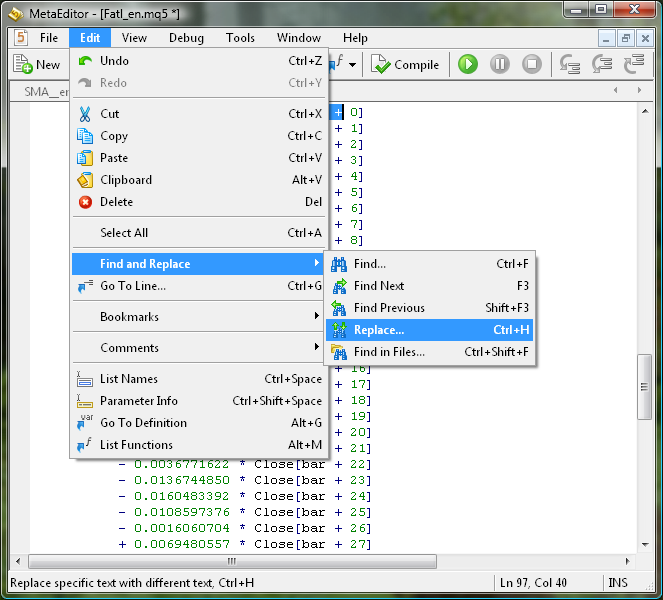The regularly met Close [bar + expression should be replaced with price [bar -: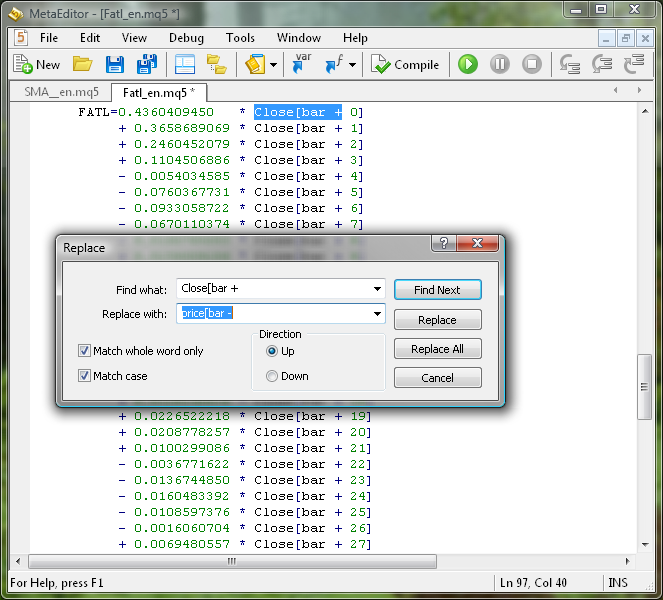In this dialog box click "Replace All" button. As a result, we obtain the required formula for FATL indicator calculation in MQL5:

```     FATL =  0.4360409450 * price[bar - 0]
+ 0.3658689069 * price[bar - 1]
+ 0.2460452079 * price[bar - 2]
+ 0.1104506886 * price[bar - 3]
- 0.0054034585 * price[bar - 4]
- 0.0760367731 * price[bar - 5]
- 0.0933058722 * price[bar - 6]
- 0.0670110374 * price[bar - 7]
- 0.0190795053 * price[bar - 8]
+ 0.0259609206 * price[bar - 9]
+ 0.0502044896 * price[bar - 10]
+ 0.0477818607 * price[bar - 11]
+ 0.0249252327 * price[bar - 12]
- 0.0047706151 * price[bar - 13]
- 0.0272432537 * price[bar - 14]
- 0.0338917071 * price[bar - 15]
- 0.0244141482 * price[bar - 16]
- 0.0055774838 * price[bar - 17]
+ 0.0128149838 * price[bar - 18]
+ 0.0226522218 * price[bar - 19]
+ 0.0208778257 * price[bar - 20]
+ 0.0100299086 * price[bar - 21]
- 0.0036771622 * price[bar - 22]
- 0.0136744850 * price[bar - 23]
- 0.0160483392 * price[bar - 24]
- 0.0108597376 * price[bar - 25]
- 0.0016060704 * price[bar - 26]
+ 0.0069480557 * price[bar - 27]
+ 0.0110573605 * price[bar - 28]
+ 0.0095711419 * price[bar - 29]
+ 0.0040444064 * price[bar - 30]
- 0.0023824623 * price[bar - 31]
- 0.0067093714 * price[bar - 32]
- 0.0072003400 * price[bar - 33]
- 0.0047717710 * price[bar - 34]
+ 0.0005541115 * price[bar - 35]
+ 0.0007860160 * price[bar - 36]
+ 0.0130129076 * price[bar - 37]
+ 0.0040364019 * price[bar - 38];```

Now we can start to code the indicator, which calculation algorithm has just been considered. To do this, first of all open SMA_1_en.mq5 indicator in MetaEditor and save it as FATL_en.mq5. Indicator template is ready, and now we have to replace the indicator calculation algorithm in it and to make some changes in variables, mostly cosmetic. You should select the entire block of the last mentioned formula for FATL filter calculation, and copy it to Windows clipboard. Then, now in the FATL.mq5 indicator code, remove all the code inside the loop operator, except the last initialization of the indicator buffer:

```//---- Main loop of indicator calculation
for(bar=first; bar<rates_total; bar++)
{

//---- Indicator buffer's cell initialization with FATL value
ExtLineBuffer[bar]=FATL;
}```

Instead of this deleted code, we'll paste the FATL digital filter calculation algorithm from Windows clipboard. Then we should replace the SMA word with more appropriate FATL, using the replacement procedure described by me above. Absolutely the same, we should replace the names of MAPeriod and MAShift input variables with FATLPeriod and FATLShft respectively. The FATLPeriod variable should be removed from the external variables, because it has a fixed value equal to 39. For the same reason, it should be removed from StringConcatenate() operator in OnInit() function. Now there is no need in the iii local variable, so it can be removed. And finally, you can change the color of indicator line to blue and make the line itself a bit thicker.

After these simple manipulation with SMA_1_en.mq5 code we get the desired indicator code FATL_en.mq5:

```//+------------------------------------------------------------------+
//|                                                      Fatl_en.mq5 |
//|                        Copyright 2010, MetaQuotes Software Corp. |
//|                                              https://www.mql5.com |
//+------------------------------------------------------------------+
//---- Indicator's author
#property copyright "2010, MetaQuotes Software Corp."
//---- Indicator version number
#property version   "1.00"
//---- Drawing the indicator in the main window
#property indicator_chart_window
//---- One buffer is used for calculating and drawing the indicator
#property indicator_buffers 1
//---- Only one graphical plotting is used
#property indicator_plots   1
//---- Drawing the indicator as line
#property indicator_type1   DRAW_LINE
//---- Blue is used as indicator's line color
#property indicator_color1  Blue
//---- Indicator line is continuous curve
#property indicator_style1  STYLE_SOLID
//---- Indicator line thickness is equal to 2
#property indicator_width1  2
//---- Displaying indicator's label
#property indicator_label1  "FATL"

//---- Input parameters of indicator
input int FATLShift=0; // FATL horizontal shift in bars

//---- Declaring and initializing a variable to store the number of calculated bars
int FATLPeriod=39;

//---- Declaration of dynamic array, which will be
//     used later as indicator buffer
double ExtLineBuffer[];
//+------------------------------------------------------------------+
//| Custom indicator initialization function                         |
//+------------------------------------------------------------------+
void OnInit()
{
//----+
//---- Transformation of ExtLineBuffer dynamic array into indicator buffer
SetIndexBuffer(0,ExtLineBuffer,INDICATOR_DATA);
//---- Horizontal shift of indicator by FATLShift
PlotIndexSetInteger(0,PLOT_SHIFT,FATLShift);
//---- Setting the position from which the drawing of indicator will start
PlotIndexSetInteger(0,PLOT_DRAW_BEGIN,FATLPeriod);
//---- Variable initialization for indicator's short name
string shortname;
StringConcatenate(shortname,"FATL(",FATLShift,")");
//--- Creating labels to display in Data Window
PlotIndexSetString(0,PLOT_LABEL,shortname);
//--- Creating name to display in a separate window and in tool-tip
IndicatorSetString(INDICATOR_SHORTNAME,shortname);
//--- Defining accuracy of displaying indicator's values
IndicatorSetInteger(INDICATOR_DIGITS,_Digits+1);
//--- Prohibition of displaying blank values
PlotIndexSetDouble(0,PLOT_EMPTY_VALUE,0.0);
//----+
}
//+------------------------------------------------------------------+
//| Custom indicator iteration function                              |
//+------------------------------------------------------------------+
int OnCalculate(
const int rates_total,     // amount of history in bars at the current tick
const int prev_calculated, // amount of history in bars at the previous tick
const int begin,           // beginning number of reliable count of bars
const double &price[]      // price array for indicator calculation
)
{
//----+
//---- Check if the number of bars is sufficient for calculation
if(rates_total<FATLPeriod-1+begin)
return(0);

//---- Declaring local variables
int first,bar;
double Sum,FATL;

//---- Calculating the 'first' starting number for the bars recalculation loop
if(prev_calculated==0)        // Checking the first start of the indicator calculation
{
first=FATLPeriod-1+begin;  // Starting number for calculation of all bars
//--- Increasing the start of data position by 'begin' bars, because the
//    calculations are based on data of another indicator
if(begin>0)
PlotIndexSetInteger(0,PLOT_DRAW_BEGIN,begin+FATLPeriod);
}
else first=prev_calculated-1; // Starting number for calculation of new bars

//---- Main loop of indicator calculation
for(bar=first; bar<rates_total; bar++)
{
//----
FATL=0.4360409450*price[bar-0]
+ 0.3658689069 * price[bar - 1]
+ 0.2460452079 * price[bar - 2]
+ 0.1104506886 * price[bar - 3]
- 0.0054034585 * price[bar - 4]
- 0.0760367731 * price[bar - 5]
- 0.0933058722 * price[bar - 6]
- 0.0670110374 * price[bar - 7]
- 0.0190795053 * price[bar - 8]
+ 0.0259609206 * price[bar - 9]
+ 0.0502044896 * price[bar - 10]
+ 0.0477818607 * price[bar - 11]
+ 0.0249252327 * price[bar - 12]
- 0.0047706151 * price[bar - 13]
- 0.0272432537 * price[bar - 14]
- 0.0338917071 * price[bar - 15]
- 0.0244141482 * price[bar - 16]
- 0.0055774838 * price[bar - 17]
+ 0.0128149838 * price[bar - 18]
+ 0.0226522218 * price[bar - 19]
+ 0.0208778257 * price[bar - 20]
+ 0.0100299086 * price[bar - 21]
- 0.0036771622 * price[bar - 22]
- 0.0136744850 * price[bar - 23]
- 0.0160483392 * price[bar - 24]
- 0.0108597376 * price[bar - 25]
- 0.0016060704 * price[bar - 26]
+ 0.0069480557 * price[bar - 27]
+ 0.0110573605 * price[bar - 28]
+ 0.0095711419 * price[bar - 29]
+ 0.0040444064 * price[bar - 30]
- 0.0023824623 * price[bar - 31]
- 0.0067093714 * price[bar - 32]
- 0.0072003400 * price[bar - 33]
- 0.0047717710 * price[bar - 34]
+ 0.0005541115 * price[bar - 35]
+ 0.0007860160 * price[bar - 36]
+ 0.0130129076 * price[bar - 37]
+ 0.0040364019 * price[bar - 38];

//---- Indicator buffer's cell initialization with FATL value
ExtLineBuffer[bar]=FATL;
}
//----+
return(rates_total);
}
//+------------------------------------------------------------------+
```

After compiling the indicator it can be tested on chart in Client Terminal: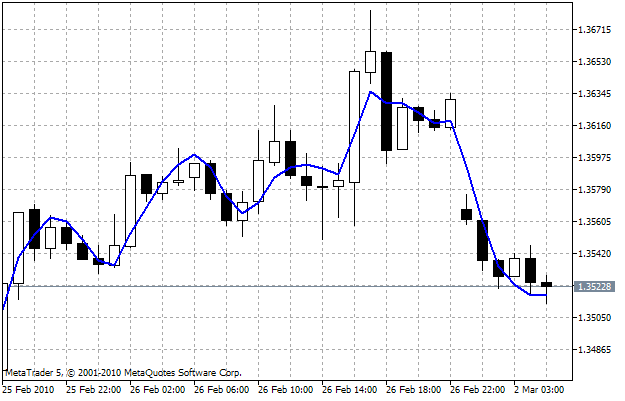It is natural, that the resulting code of FATL indicator can be used as a template for constructing other similar filters. But now the problem is much more easier. In our code it's sufficient to replace the filter calculation formula, to replace FATL word  with DIGFILTER, and to initialize (now) DIGFILTERPeriod variable with required dimension of the digital filter.

### Common solution for creating digital filters in Client Terminal

The indicator, that we've just considered, is a single variant of solving the general problem of digital signal filtering. It would be nice to have an indicator, that represents a common solution, allowing to build any digital filter using only one indicator. This problem was solved long ago for MetaTrader 4 Client Terminal using DF.dll module by Sergei Ilyuhin. So, it would be easy to use it to solve our problem in MetaTrader 5 Client Terminal. In this module the DigitalFilter() function is introduced:

`DigitalFilter(int FType, int P1, int D1, int A1, int P2, int D2, int A2, double Ripple, int Delay, double& array[]); `

It allows you to receive the digital filter coefficients as the array[] array. The function writes the digital filter coefficients into this array with size of 1500 using reference (the '&' mark after the declaration of this variable type in this array). Function accepts the values of ten input parameters and returns the size of digital filter. So, this is quite enough to build the universal digital filter. The whole problem boils down to organizing DLL import in the existing indicator on a global level, getting the array of coefficients in indicator initialization block of code, and on the basis of these coefficients running the universal calculation of filter in OnCalculate(). The input variables of DigitalFilter() function should be placed into input variables of indicator. We'll do it right now.

Importing DF.dll file doesn't cause any difficulty. It's just three lines of code:

```//---- DLL import
#import "DF.dll"
int DigitalFilter(int FType, int P1, int D1, int A1, int P2, int D2, int A2, double Ripple, int Delay, double& array[]);
#import```

After this, we'll make all external variables of DigitalFilter() function as input variables of indicator:

```//---- Input parameters of indicator
input FType_ FType=LPF;     //Filter Type
//0 - Low-Pass Filter (FATL / SATL / KGLP), 1 - High-Pass Filter (KGHP),
//2 - Band-Pass Filter (RBCI / KGBP), 3 - Band-Stop Filter (KGBS)
input int    P1 = 28;       //Cut-off period 1, in bars
input int    D1 = 19;       //Transient process cut-off period 1, in bars
input int    A1 = 40;       //Fading in delay band 1, in dB
input int    P2 = 0;        //Cut-off period 2, in bars
input int    D2 = 0;        //Transient process cut-off period 2, in bars
input int    A2 = 0;        //Fading in delay band 2, in dB
input int    Delay=0;       //Delay, in bars
input double Ripple=0.08;   //Beats in bandwidth, in dB
input int    FILTERShift=0; //Moving Average horizontal shift, in bars```

At the global level we'll declare FILTERPeriod variable without initialization:

```//---- Declaring and initializing a variable to store the number of calculated bars
int FILTERPeriod;```

At the global level we'll declare a dynamic array to store filter coefficients:

```//---- Declaration of dynamic array, which will be
//     used later as indicator buffer
double FILTERTable[];```

Now let's go into block of OnInit() function. It's not quite logical to use the FILTERTable[] array as parameter of DigitalFilter() function. For this we would make it size up to 1500 elements, of which in the OnCalculate() function block  only 100 - 200 will be used. In such situation it would be better to use locally declared Array array inside OnInit() function. Necessary amount of data from this array will be written to the FILTERTable[] array. After exiting from OnInit() function the large Array[] array will be destroyed, and the necessary data will remain in the FILTERTable[] array, which will have a size equal to the length of the FILTERPeriod digital filter. Here is the variant of code that is used for this purpose:

```//---- Calculation of digital filter coefficients and determining the size of FILTERTable[] buffer
double Array;
FILTERPeriod=DigitalFilter(FType,P1,D1,A1,P2,D2,A2,Ripple,Delay,Array);
//----  Changing the size of FILTERTable[] buffer for required number of digital filter coefficients
if(FILTERPeriod<=0)
{
Print("Input parameters are incorrect. Indicator can't operate!");
return;
}
//---- Copying data from temporary array with size of 1500 to the main array with size of FILTERPeriod
ArrayCopy(FILTERTable,Array,0,0,FILTERPeriod);```

Within the OnCalculate() function the code for filter calculation is quite simple:

```      //---- Digital filter calculation formula
FILTER=0.0;
for(iii = 0; iii<FILTERPeriod; iii++)
FILTER+= FILTERTable[iii] * price[bar - iii];```

The final version of this indicator code is presented in DFilter_en.mq5 file. The interface of this indicator can be slightly improved. The fact that the input variable of indicator takes values from 0 to 3.

```input int FType = 0; //Тип фильтра
//0 - ФНЧ (FATL/SATL/KGLP), 1 - ФВЧ (KGHP), 2 - полосовой (RBCI/KGBP), 3 - режекторный (KGBS)```

These values are much easier perceived not in numerical form, but as the names of the filter: 0 - Low-Pass Filter (FATL/SATL/KGLP), 1 - High-Pass Filter (KGHP), 2 - Band-Pass Filter (RBCI/KGBP), 3 - Band-Stop Filter (KGBS). For such a case in MQL5 there are special type of variables, called enumerations. In our case, we have to declare and initialize the enumeration before input parameters of indicator:

```//---- Declaration and initialization of digital filters types
enum FType_ //Filter Type
{
LPF, //Low-Pass Filter (FATL/SATL/KGLP)
HPF, //High-Pass Filter (KGHP)
BPF, //Band-Pass Filter (RBCI/KGBP)
BSF, //Band-Stop Filter (KGBS)
};```

After that, we have to replace the type of used variable in declaration of indicator external parameter:

`input FType_ FType = LPF; //Filter Type`

As a result, choosing the values of this parameter in indicator's dialog box looks like following: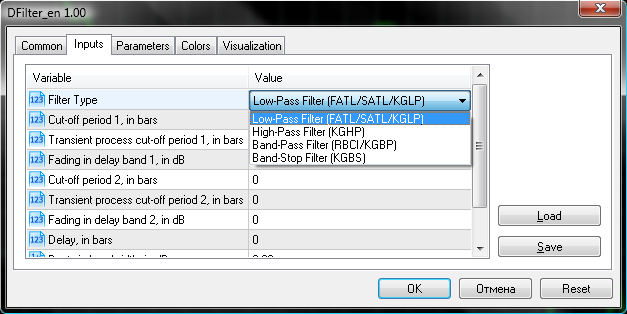As in the enumeration declaration the named constants are followed by single-line comments, then they are to be chosen as input parameters. Now we have the final version of universal digital filter source code:

```//+------------------------------------------------------------------+
//|                                                      ProjectName |
//|                                       http://www.companyname.net |
//+------------------------------------------------------------------+
/*
* <<< DIGITAL FILTERS FOR METATRADER 5 >>> *
*
* DF.dll file should be placed in "\MetaTrader 5\MQL5\Libraries\" folder.
* DF.dll requires three additional DLLs, containing a block of mathematical
* processing - bdsp.dll, lapack.dll, mkl_support.dll.
* These DLLs must be installed in "C:\Windows\System32\" folder for
* Windows 32-bit operating systems or in "C:\Windows\SysWOW64\" folder
* for Windows 64-bit operating systems.
*
* Before using, make sure that:
*
* 1. "Allow DLL import" option is enabled in Client Terminal settings
* 2. In "C:\Windows\System32\" or in "C:\Windows\SysWOW64\" folders the
*    Bdsp.dll, lapack.dll and mkl_support.dll auxiliary math libraries are present.
*
* Description of input parameters:
*
* Ftype  - Filter Type: 0 - Low-Pass Filter (FATL/SATL/KGLP), 1 - High-Pass Filter (KGHP),
*          2 - Band-Pass Filter (RBCI / KGBP), 3 - Band-Stop Filter (KGBS)
* P1     - Cut-off period P1, in bars
* D1     - Transient process cut-off period D1, in bars
* A1     - Fading in delay band A1, in dB
* P2     - Cut-off period P2, in bars
* D2     - Transient process cut-off period D2, in bars
* A2     - Fading in delay band A2, in dB
* Ripple - Beats in bandwidth, in dB
* Delay  - Delay, in bars
*
* For Low-Pass Filter and HPF the values of P2, D2, A2 are ignored
*
* Conditions:
* Low-Pass Filter:                       P1>D1
* High-Pass Filter:                      P1<D1
* Band-Pass Filter and Band-Stop Filter: D2>P2>P1>D1
*/
//+------------------------------------------------------------------+
//|      Digital Low Pass (FATL/SATL, KGLP) Filter    DFilter_en.mq5 |
//|                    Digital Filter: Copyright (c) Sergey Ilyukhin |
//|                           Moscow, qpo@mail.ru  http://fx.qrz.ru/ |
//|                              MQL5 CODE: 2010,   Nikolay Kositsin |
//|                              Khabarovsk,   farria@mail.redcom.ru |
//+------------------------------------------------------------------+
//---- Indicator's author
#property copyright "2005, Sergey Ilyukhin, Moscow"
//---- Indicator version number
#property version   "1.00"
//---- Drawing the indicator in main window
#property indicator_chart_window
//---- One buffer is used for calculating and drawing the indicator
#property indicator_buffers 1
//---- Only one graphical plotting is used
#property indicator_plots   1
//---- Drawing the indicator as line
#property indicator_type1   DRAW_LINE
//---- Blue is used as indicator's line color
#property indicator_color1  DarkViolet
//---- Indicator line is continuous curve
#property indicator_style1  STYLE_SOLID
//---- Indicator line thickness is equal to 2
#property indicator_width1  2
//---- Displaying indicator's label
#property indicator_label1  "DFilter"
//---- Declaration and initialization of digital filters types
enum FType_ //Filter Type
{
LPF, //Low-Pass Filter (FATL/SATL/KGLP)
HPF, //High-Pass Filter (KGHP)
BPF, //Band-Pass Filter (RBCI/KGBP)
BSF, //Band-Stop Filter (KGBS)
};

//---- Input parameters of indicator
input FType_ FType=LPF;     //Filter Type
//0 - Low-Pass Filter (FATL / SATL / KGLP), 1 - High-Pass Filter (KGHP),
//2 - Band-Pass Filter (RBCI / KGBP), 3 - Band-Stop Filter (KGBS)
input int    P1 = 28;       //Cut-off period 1, in bars
input int    D1 = 19;       //Transient process cut-off period 1, in bars
input int    A1 = 40;       //Fading in delay band 1, in dB
input int    P2 = 0;        //Cut-off period 2, in bars
input int    D2 = 0;        //Transient process cut-off period 2, in bars
input int    A2 = 0;        //Fading in delay band 2, in dB
input int    Delay=0;       //Delay, in bars
input double Ripple=0.08;   //Beats in bandwidth, in dB
input int    FILTERShift=0; //Moving Average horizontal shift, in bars

//---- DLL Import
#import "DF.dll"
int DigitalFilter(int FType,int P1,int D1,int A1,int P2,int D2,int A2,double Ripple,int Delay,double &array[]);
#import

//---- Declaring and initializing a variable to store the number of calculated bars
int FILTERPeriod;

//---- Declaration of dynamic array, which will be
//     used later as indicator buffer
double ExtLineBuffer[];

//---- Declaring and initializing an array for the digital filter coefficients
double FILTERTable[];
//+------------------------------------------------------------------+
//| Custom indicator initialization function                         |
//+------------------------------------------------------------------+
void OnInit()
{
//----+
//---- Transformation of ExtLineBuffer dynamic array into indicator buffer
SetIndexBuffer(0,ExtLineBuffer,INDICATOR_DATA);
//---- Horizontal shift of indicator by FILTERShift
PlotIndexSetInteger(0,PLOT_SHIFT,FILTERShift);
//---- Setting the position from which the drawing of indicator will start
PlotIndexSetInteger(0,PLOT_DRAW_BEGIN,FILTERPeriod);
//---- Variable initialization for indicator's short name
string shortname;
StringConcatenate(shortname,"FILTER(",FILTERShift,")");
//---- Creating label to display in Data Window
PlotIndexSetString(0,PLOT_LABEL,shortname);
//---- Creating name to display in a separate window and in tool-tip
IndicatorSetString(INDICATOR_SHORTNAME,shortname);
//---- Defining accuracy of displaying indicator's values
IndicatorSetInteger(INDICATOR_DIGITS,_Digits+1);
//---- Prohibition of empty values plotting
PlotIndexSetDouble(0,PLOT_EMPTY_VALUE,0.0);
//---- Calculation of digital filter coefficients and determining the size of FILTERTable[] buffer
double Array;
FILTERPeriod=DigitalFilter(FType,P1,D1,A1,P2,D2,A2,Ripple,Delay,Array);
//----  Changing the size of FILTERTable[] buffer for required number of digital filter coefficients
if(FILTERPeriod<=0)
{
Print("Input parameters are incorrect. Indicator can't operate!");
return;
}
//---- Copying data from temporary array with size of 1500 to the main array with size of FILTERPeriod
ArrayCopy(FILTERTable,Array,0,0,FILTERPeriod);
//----+
}
//+------------------------------------------------------------------+
//| Custom indicator iteration function                              |
//+------------------------------------------------------------------+
int OnCalculate(
const int rates_total,     // amount of history in bars at the current tick
const int prev_calculated, // amount of history in bars at the previous tick
const int begin,           // beginning number of reliable count of bars
const double &price[]      // price array for indicator calculation
)
{
//----+
//---- Check if the number of bars is sufficient for calculation
if(rates_total<FILTERPeriod-1+begin)
return(0);

//---- Declaring local variables
int first,bar,iii;
double Sum,FILTER;

//---- Calculating the 'first' starting number for the bars recalculation loop
if(prev_calculated==0)         // Checking the first start of the indicator calculation
{
first=FILTERPeriod-1+begin; // Starting number for calculation of all bars
//---- Increasing the start of data position by 'begin' bars,
//     because the calculations are based on data of another indicator
if(begin>0)
PlotIndexSetInteger(0,PLOT_DRAW_BEGIN,begin+FILTERPeriod);
}
else first=prev_calculated-1;  // Starting number for calculation of new bars

//---- Main loop of indicator calculation
for(bar=first; bar<rates_total; bar++)
{
//---- Digital filter calculation formula
FILTER=0.0;
for(iii = 0; iii<FILTERPeriod; iii++)
FILTER+= FILTERTable[iii] * price[bar - iii];

//---- Indicator buffer's cell initialization with FILTER value
ExtLineBuffer[bar]=FILTER;
}
//----+
return(rates_total);
}
//+------------------------------------------------------------------+
```
The MQL5 implementation of such universal digital filter just by means of Client Terminal completely closes the need for any digital filter from FinWare company. This is a significant convenience, which opens up new possibilities in using these indicators.

### Conclusion

After all these manipulations with code it obtained a lot of details. But at a closer look at these details of this process, everything works perfectly logical and understandable, if we begin with analysis of most simple things and continue to make meaningful and deliberate transition from simple to complex.

Translated from Russian by MetaQuotes Software Corp.
Original article: https://www.mql5.com/ru/articles/32

Attached files |
dll.rar (1302.47 KB)
sma__en.mq5 (3.56 KB)
sma_1_en.mq5 (4.51 KB)
fatl_en.mq5 (6.08 KB)
dfilter_0_en.mq5 (8.17 KB)
dfilter_en.mq5 (8.42 KB)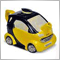MQL5 for Newbies: Guide to Using Technical Indicators in Expert Advisors

In order to obtain values of a built-in or custom indicator in an Expert Advisor, first its handle should be created using the corresponding function. Examples in the article show how to use this or that technical indicator while creating your own programs. The article describes indicators that are built n the MQL5 language. It is intended for those who don't have much experience in the development of trading strategies and offers simple and clear ways of working with indicators using the offered library of functions.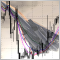Event handling in MQL5: Changing MA period on-the-fly

Suppose that simple MA (Moving Average) indicator with period 13 is applied to a chart. And we want to change the period to 20, but we do not want to go to indicator properties dialog box and edit the number 13 to 20: simply tired of these tedious actions with mouse and keyboard. And especially we don't want to open indicator code and modify it. We want to do all this with a single press of a button - "up arrows" next to the numeric keypad. In this article I'll describe how to do it.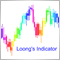The Drawing Styles in MQL5

There are 6 drawing styles in MQL4 and 18 drawing styles in MQL5. Therefore, it may be worth writing an article to introduce MQL5's drawing styles. In this article, we will consider the details of drawing styles in MQL5. In addition, we will create an indicator to demonstrate how to use these drawing styles, and refine the plotting.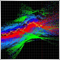Drawing Indicator's Emissions in MQL5

In this article, we will consider the emission of indicators - a new approach to the market research. The calculation of emission is based on the intersection of different indicators: more and more points with different colors and shapes appear after each tick. They form numerous clusters like nebulae, clouds, tracks, lines, arcs, etc. These shapes help to detect the invisible springs and forces that affect the movement of market prices.